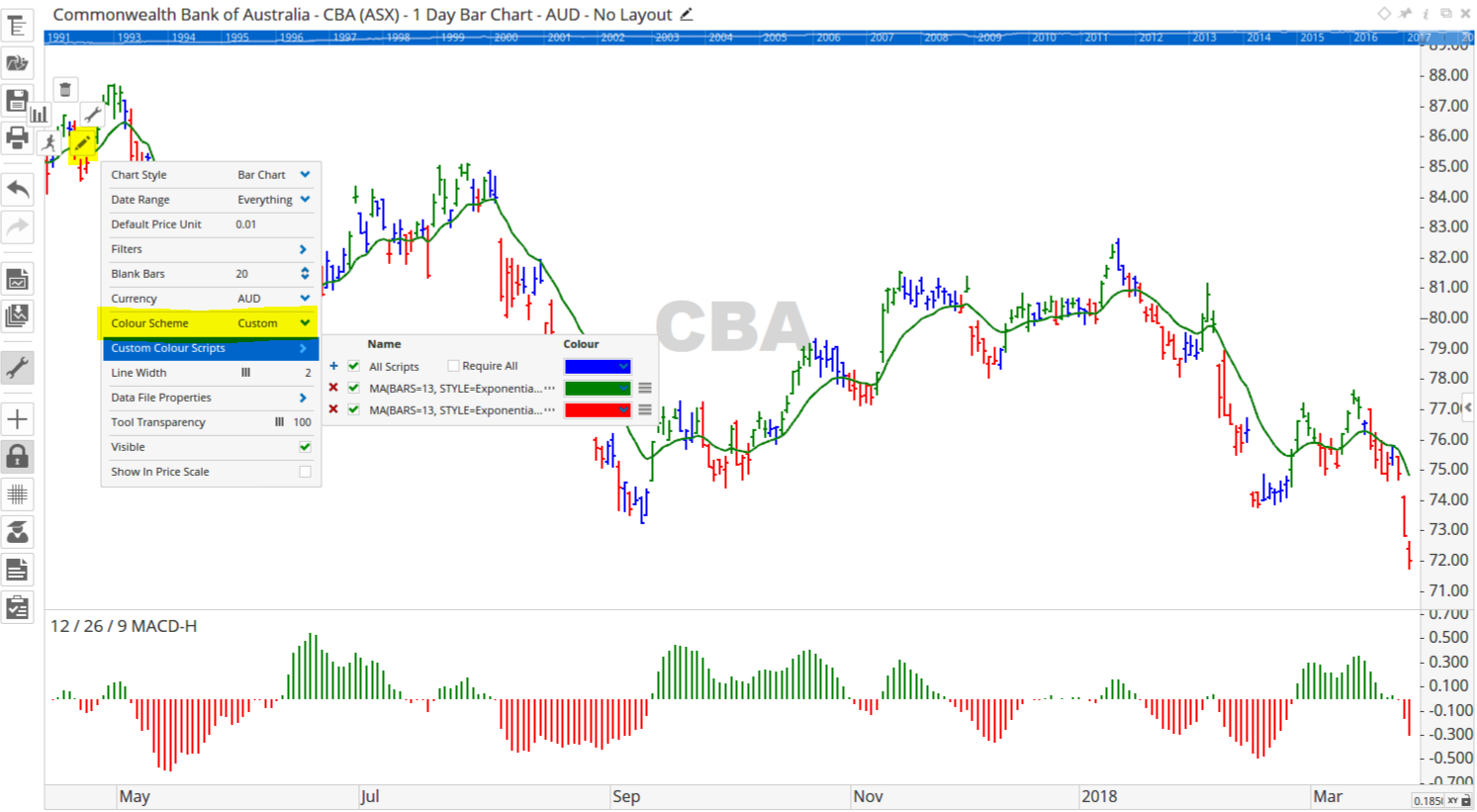Select Page

# Reply To: Impulse system colouring of bars

Optuma Forums Optuma Scripting Impulse system colouring of bars Reply To: Impulse system colouring of bars

#45699

Hi Peter,

Is this the formula you need?

Green Bars: 13-period EMA rising and MACD(12,26,9) histogram rising
Red Bars: 13-period EMA falling and MACD(12,26,9) histogram falling
Blue Bars: when neither above is true

You can create a custom colour scheme using the following formulas, with blue selected as the default colour:

Green Bars: MA(BARS=13, STYLE=Exponential, CALC=Close) IsUp and MACD(BAR1=12, BAR2=26, OSC=9) IsUp
Red Bars: MA(BARS=13, STYLE=Exponential, CALC=Close) IsDown and MACD(BAR1=12, BAR2=26, OSC=9) IsDown